# ML Aggarwal Solutions for Class 8 Maths Chapter 2 - Exponents and Powers

ML Aggarwal Solutions for Class 8 Maths Chapter 2 – Exponents and Powers are provided here to help students prepare for their exams and score well. This chapter mainly deals with problems based on exponents and its powers. To help students analyze their problem-solving ability the concepts are explained in a step by step format by the experienced faculty team at BYJU’S, having vast knowledge about the concepts. Students are advised to practice on a regular basis, which helps them crack difficult questions at ease in their examinations. ML Aggarwal Solutions book is one of the best study materials one can opt for their exam preparation, which yields good results. The solutions PDF is made readily available for students to download easily from the links given below and can start practising offline as well.

Chapter 2 – Exponents and Powers contains two exercises and the ML Aggarwal Class 8 Solutions present in this page provides solutions to questions related to each exercise present in this chapter.

## Download the Pdf of ML Aggarwal Solutions for Class 8 Maths Chapter 2 – Exponents and Powers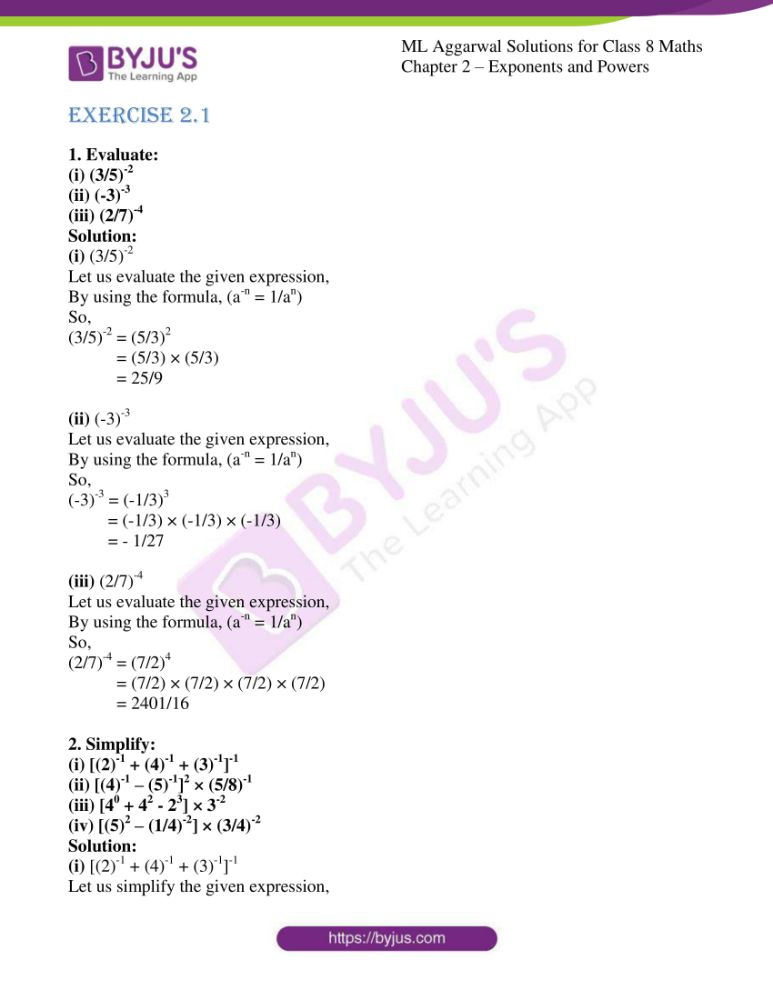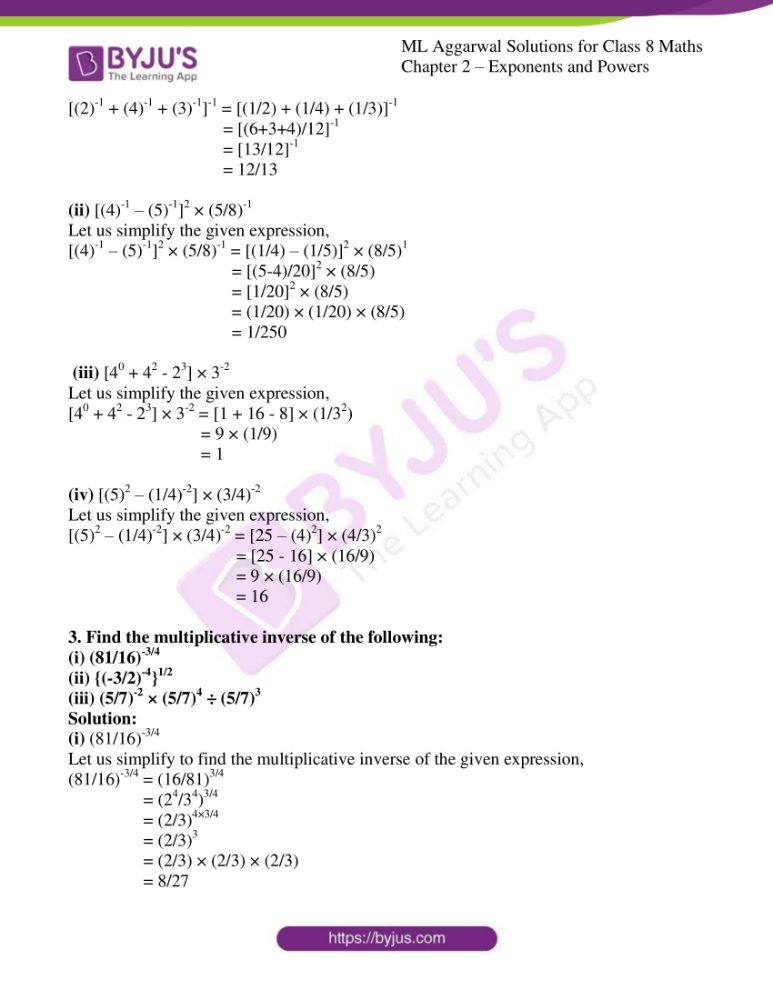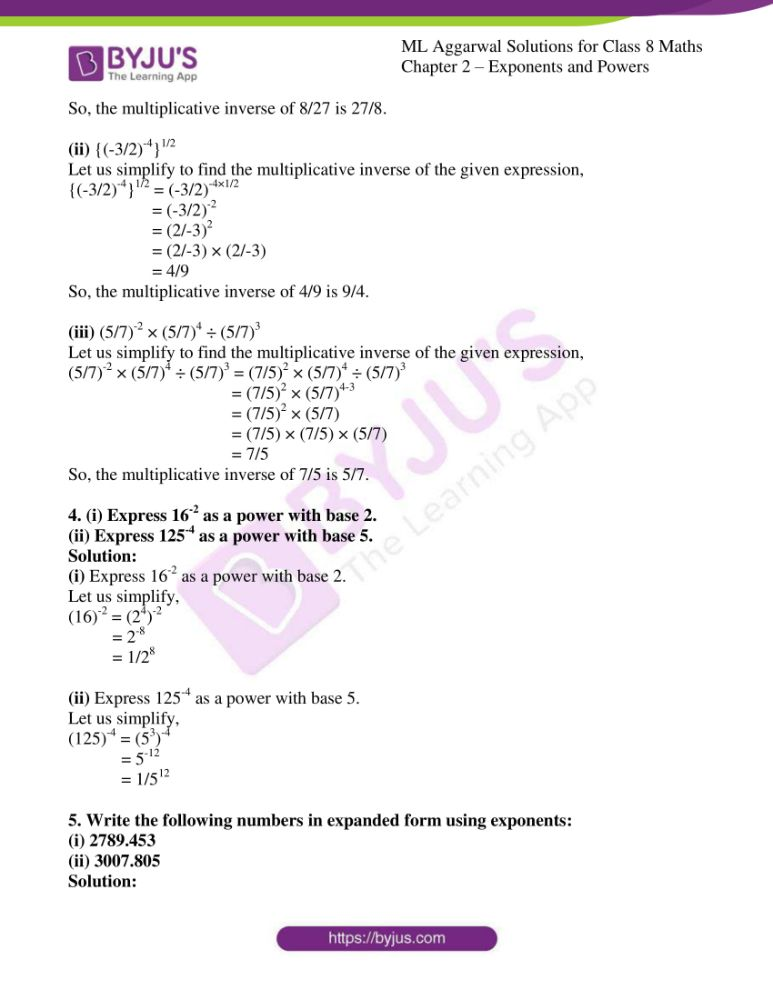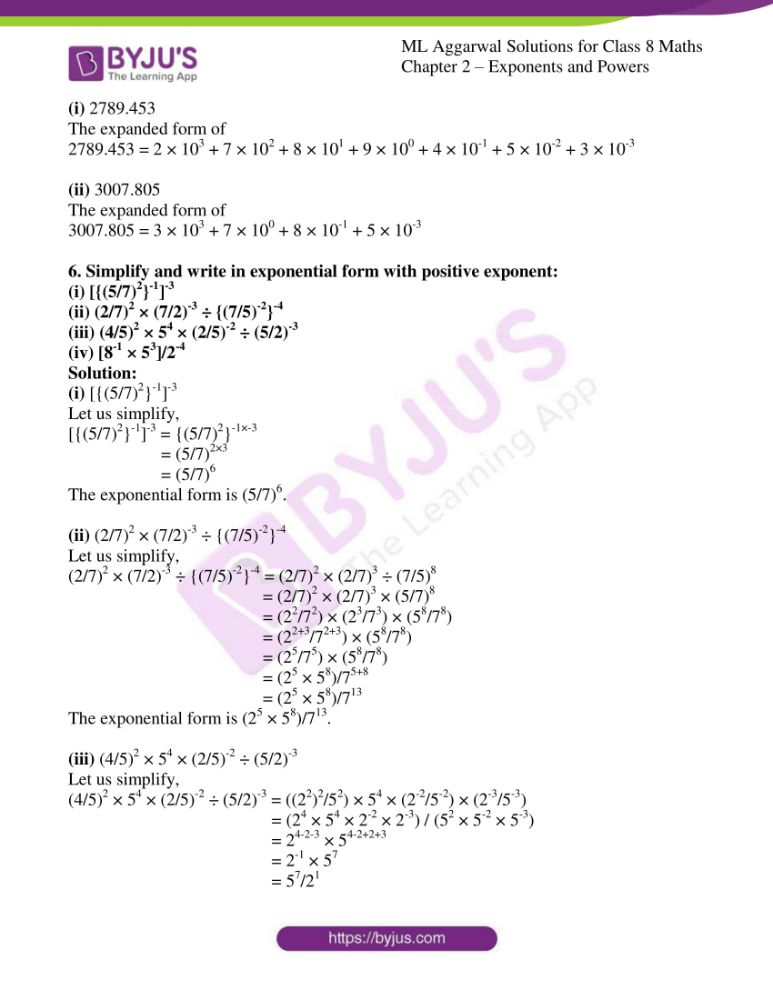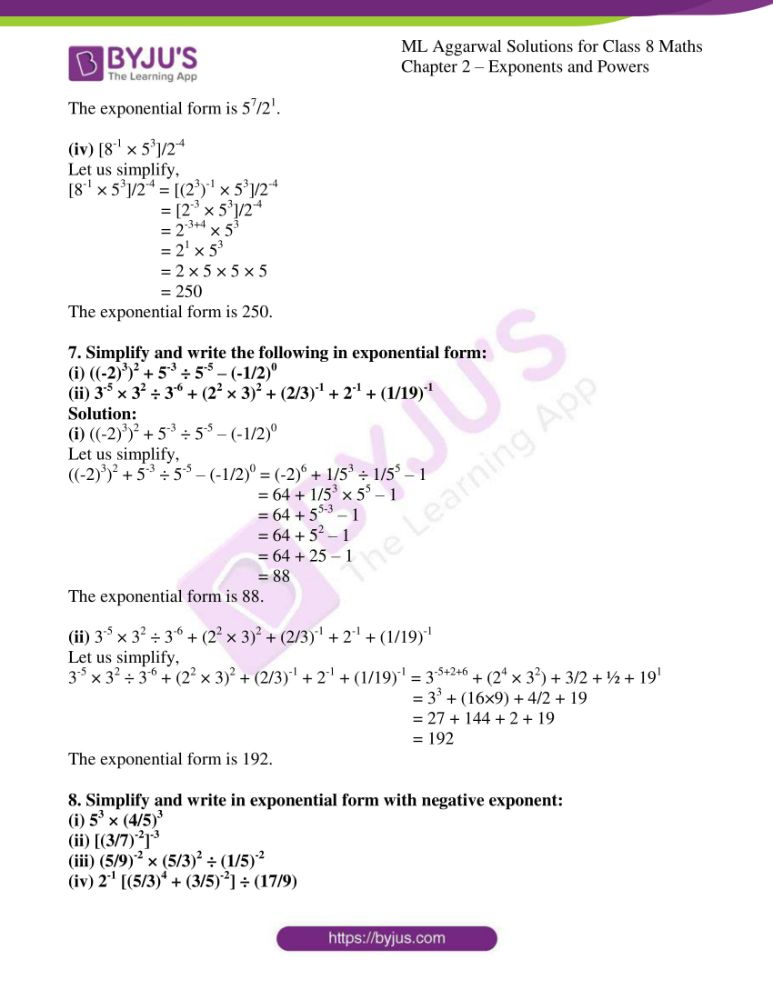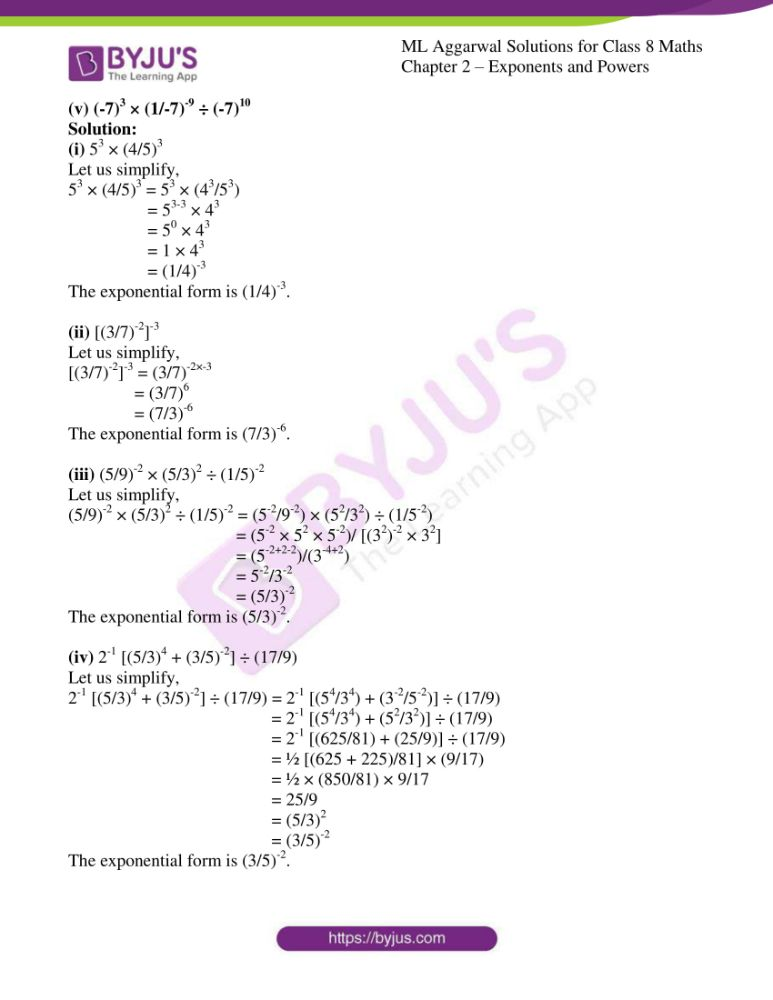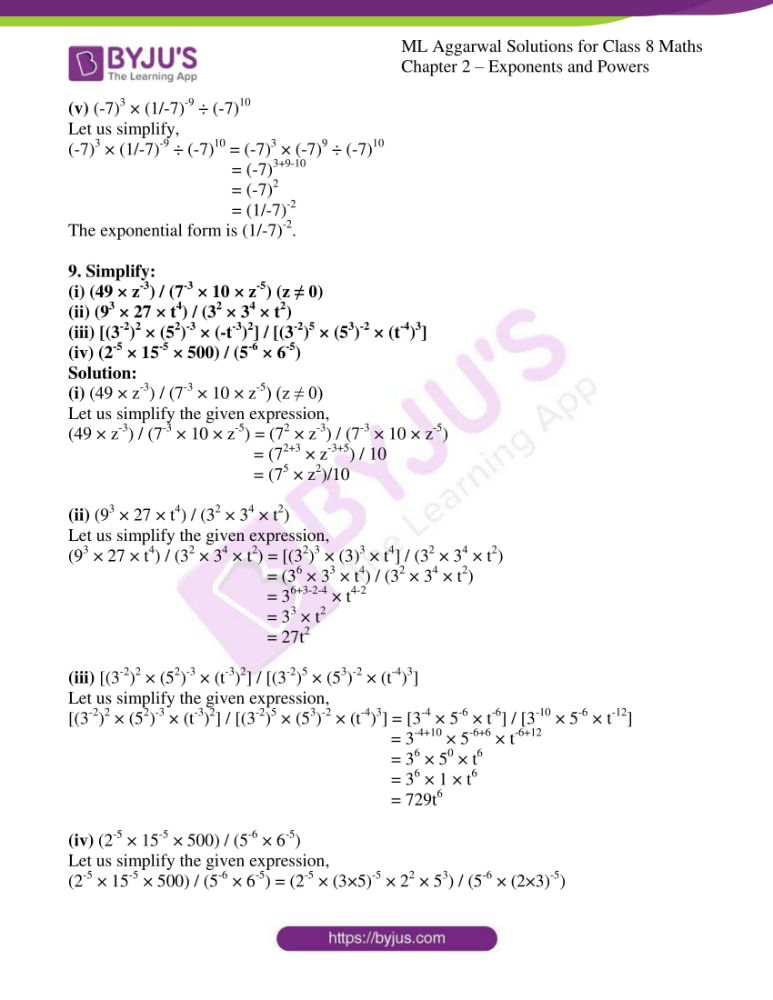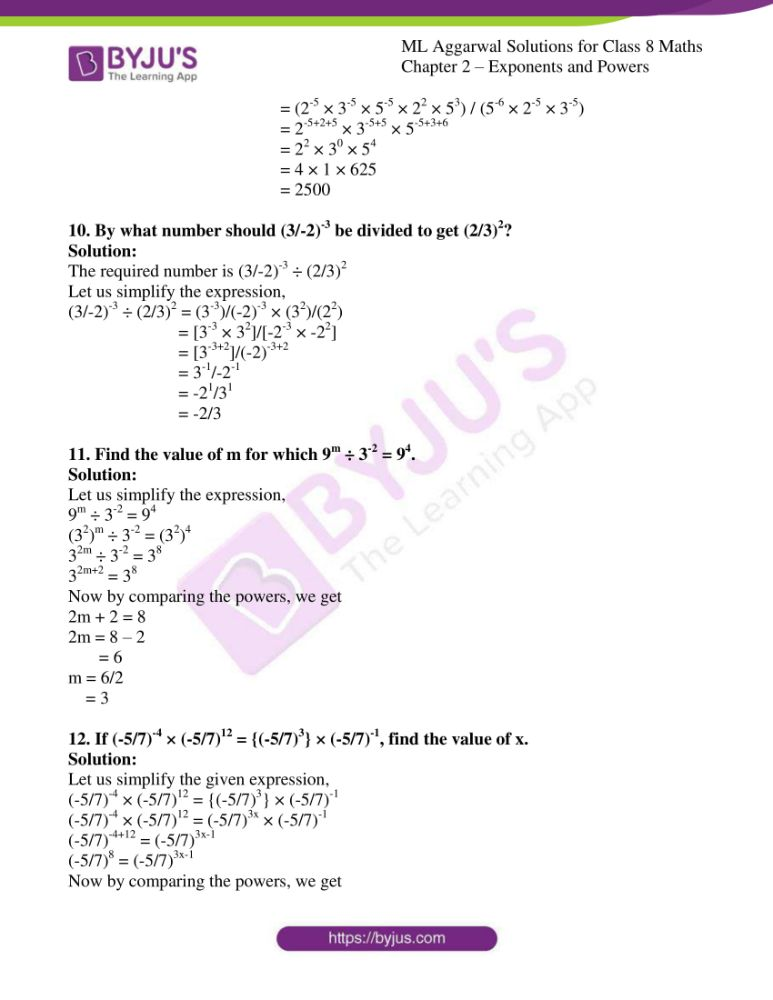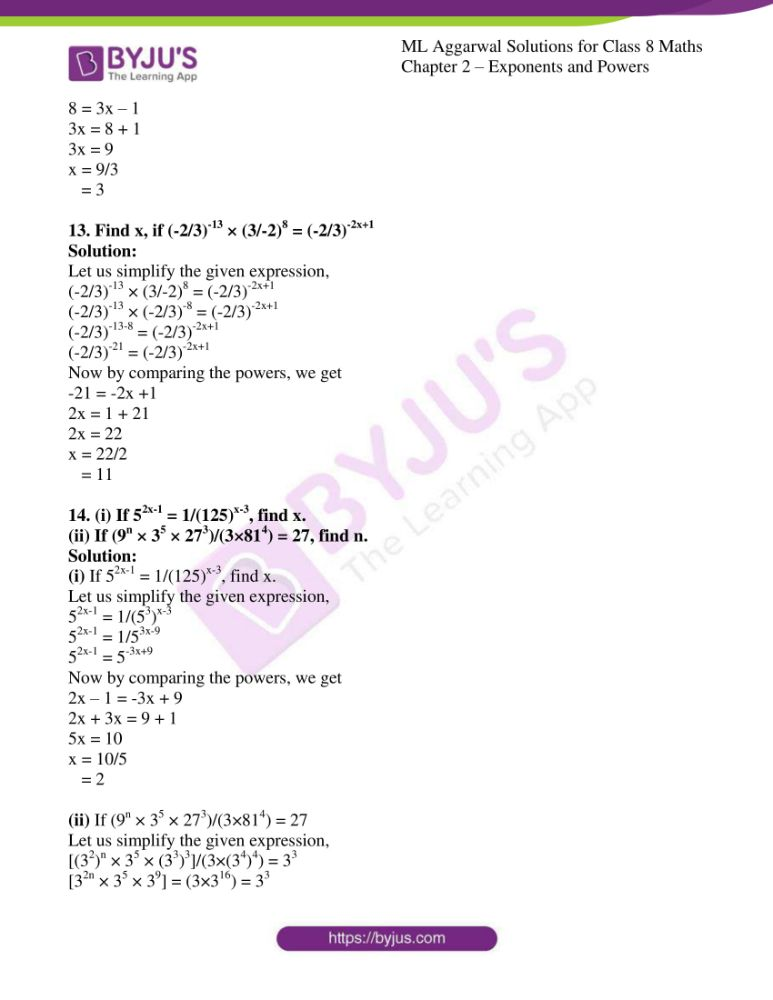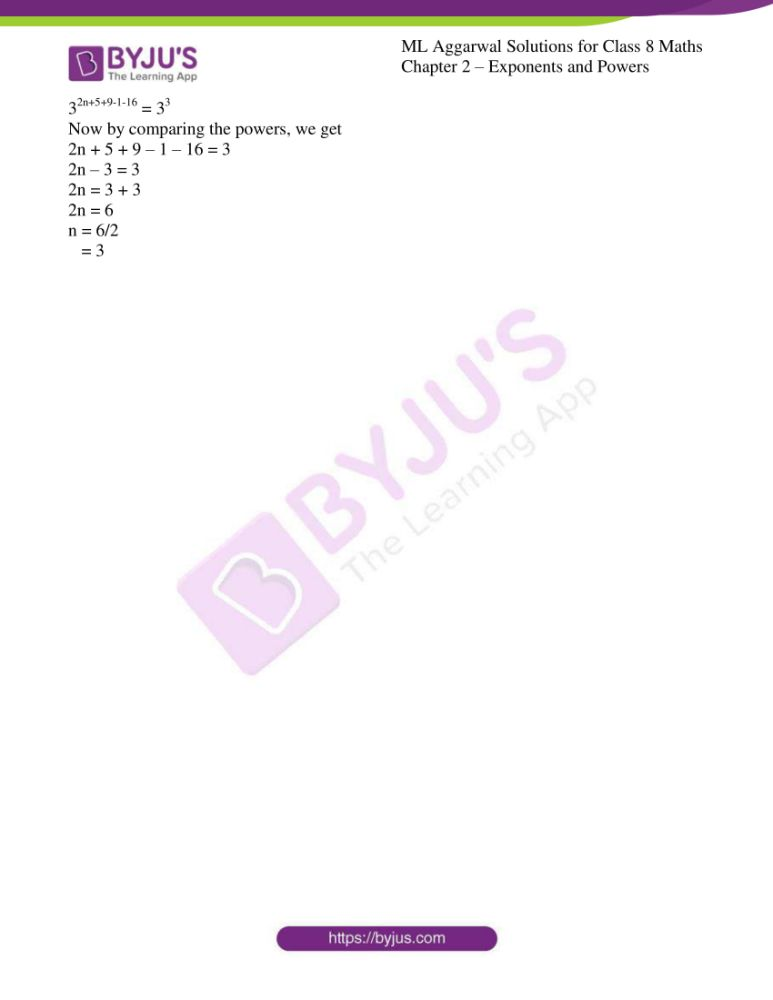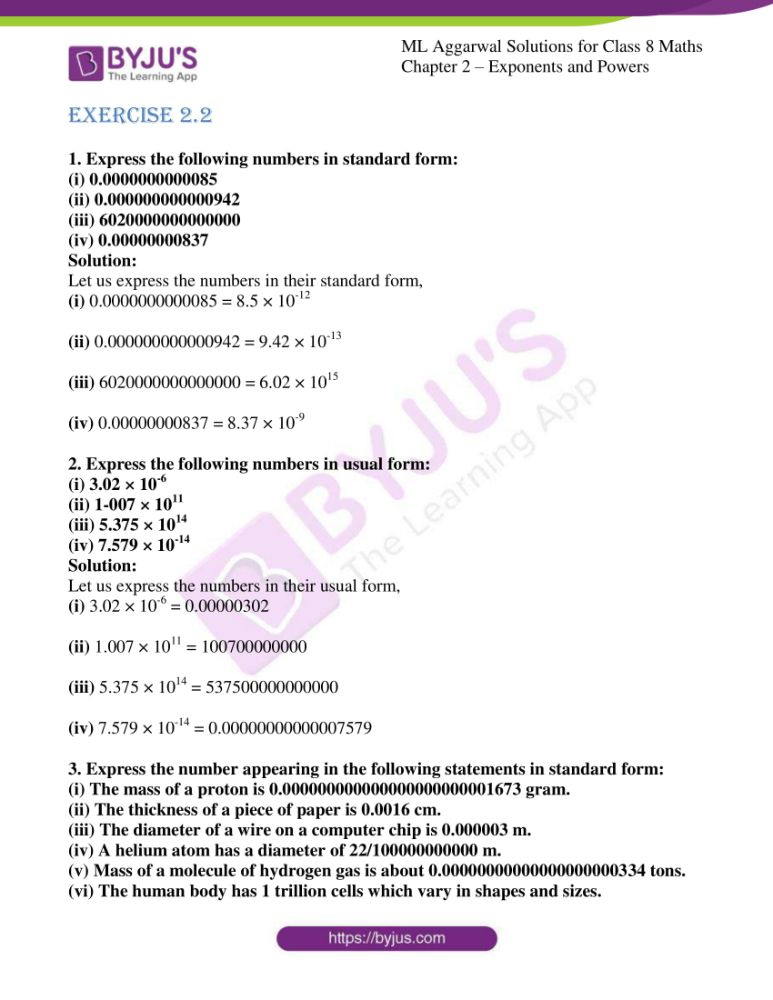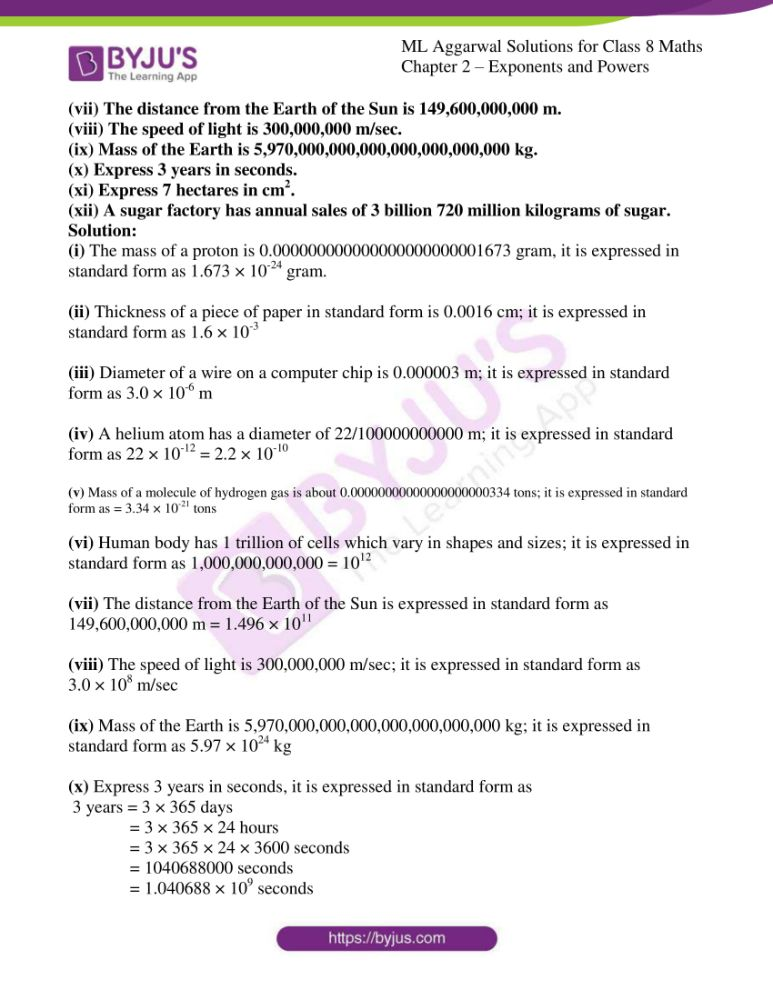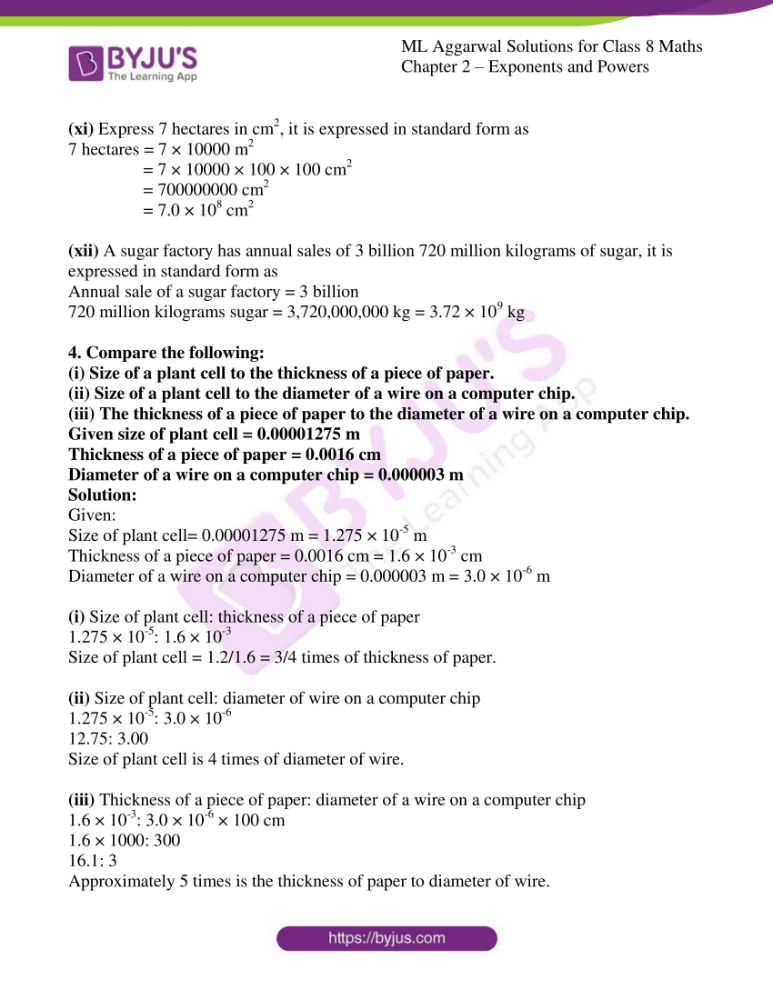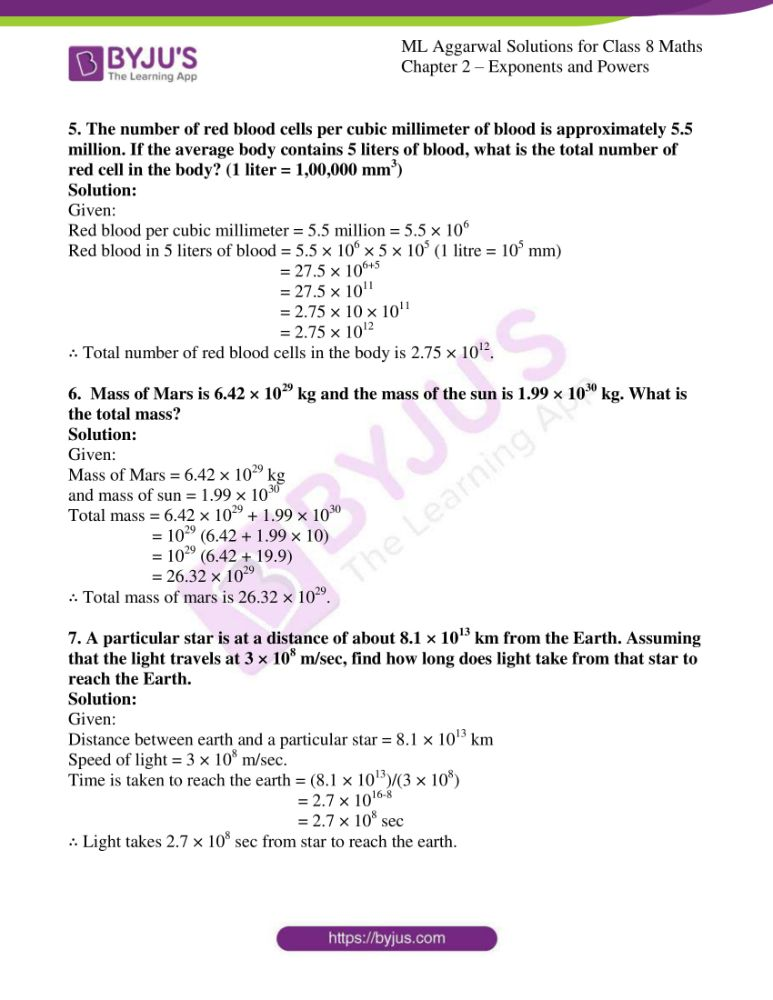### Access answers to ML Aggarwal Solutions for Class 8 Maths Chapter 2 – Exponents and Powers

EXERCISE 2.1

1. Evaluate:

(i) (3/5)-2

(ii) (-3)-3

(iii) (2/7)-4

Solution:

(i) (3/5)-2

Let us evaluate the given expression,

By using the formula, (a-n = 1/an)

So,

(3/5)-2 = (5/3)2

= (5/3) × (5/3)

= 25/9

(ii) (-3)-3

Let us evaluate the given expression,

By using the formula, (a-n = 1/an)

So,

(-3)-3 = (-1/3)3

= (-1/3) × (-1/3) × (-1/3)

= – 1/27

(iii) (2/7)-4

Let us evaluate the given expression,

By using the formula, (a-n = 1/an)

So,

(2/7)-4 = (7/2)4

= (7/2) × (7/2) × (7/2) × (7/2)

= 2401/16

2. Simplify:

(i) [(2)-1 + (4)-1 + (3)-1]-1

(ii) [(4)-1 – (5)-1]2 × (5/8)-1

(iii) [40 + 42 – 23] × 3-2

(iv) [(5)2 – (1/4)-2] × (3/4)-2

Solution:

(i) [(2)-1 + (4)-1 + (3)-1]-1

Let us simplify the given expression,

[(2)-1 + (4)-1 + (3)-1]-1 = [(1/2) + (1/4) + (1/3)]-1

= [(6+3+4)/12]-1

= [13/12]-1

= 12/13

(ii) [(4)-1 – (5)-1]2 × (5/8)-1

Let us simplify the given expression,

[(4)-1 – (5)-1]2 × (5/8)-1 = [(1/4) – (1/5)]2 × (8/5)1

= [(5-4)/20]2 × (8/5)

= [1/20]2 × (8/5)

= (1/20) × (1/20) × (8/5)

= 1/250

(iii) [40 + 42 – 23] × 3-2

Let us simplify the given expression,

[40 + 42 – 23] × 3-2 = [1 + 16 – 8] × (1/32)

= 9 × (1/9)

= 1

(iv) [(5)2 – (1/4)-2] × (3/4)-2

Let us simplify the given expression,

[(5)2 – (1/4)-2] × (3/4)-2 = [25 – (4)2] × (4/3)2

= [25 – 16] × (16/9)

= 9 × (16/9)

= 16

3. Find the multiplicative inverse of the following:

(i) (81/16)-3/4

(ii) {(-3/2)-4}1/2

(iii) (5/7)-2 × (5/7)4 ÷ (5/7)3

Solution:

(i) (81/16)-3/4

Let us simplify to find the multiplicative inverse of the given expression,

(81/16)-3/4 = (16/81)3/4

= (24/34)3/4

= (2/3)4×3/4

= (2/3)3

= (2/3) × (2/3) × (2/3)

= 8/27

So, the multiplicative inverse of 8/27 is 27/8.

(ii) {(-3/2)-4}1/2

Let us simplify to find the multiplicative inverse of the given expression,

{(-3/2)-4}1/2 = (-3/2)-4×1/2

= (-3/2)-2

= (2/-3)2

= (2/-3) × (2/-3)

= 4/9

So, the multiplicative inverse of 4/9 is 9/4.

(iii) (5/7)-2 × (5/7)4 ÷ (5/7)3

Let us simplify to find the multiplicative inverse of the given expression,

(5/7)-2 × (5/7)4 ÷ (5/7)3 = (7/5)2 × (5/7)4 ÷ (5/7)3

= (7/5)2 × (5/7)4-3

= (7/5)2 × (5/7)

= (7/5) × (7/5) × (5/7)

= 7/5

So, the multiplicative inverse of 7/5 is 5/7.

4. (i) Express 16-2 as a power with base 2.
(ii) Express 125-4 as a power with base 5.
Solution:

(i) Express 16-2 as a power with base 2.

Let us simplify,

(16)-2 = (24)-2

= 2-8

= 1/28

(ii) Express 125-4 as a power with base 5.

Let us simplify,

(125)-4 = (53)-4

= 5-12

= 1/512

5. Write the following numbers in expanded form using exponents:
(i) 2789.453
(ii) 3007.805
Solution:

(i) 2789.453

The expanded form of

2789.453 = 2 × 103 + 7 × 102 + 8 × 101 + 9 × 100 + 4 × 10-1 + 5 × 10-2 + 3 × 10-3

(ii) 3007.805

The expanded form of

3007.805 = 3 × 103 + 7 × 100 + 8 × 10-1 + 5 × 10-3

6. Simplify and write in exponential form with positive exponent:

(i) [{(5/7)2}-1]-3

(ii) (2/7)2 × (7/2)-3 ÷ {(7/5)-2}-4

(iii) (4/5)2 × 54 × (2/5)-2 ÷ (5/2)-3

(iv) [8-1 × 53]/2-4

Solution:

(i) [{(5/7)2}-1]-3

Let us simplify,

[{(5/7)2}-1]-3 = {(5/7)2}-1×-3

= (5/7)2×3

= (5/7)6

The exponential form is (5/7)6.

(ii) (2/7)2 × (7/2)-3 ÷ {(7/5)-2}-4

Let us simplify,

(2/7)2 × (7/2)-3 ÷ {(7/5)-2}-4 = (2/7)2 × (2/7)3 ÷ (7/5)8

= (2/7)2 × (2/7)3 × (5/7)8

= (22/72) × (23/73) × (58/78)

= (22+3/72+3) × (58/78)

= (25/75) × (58/78)

= (25 × 58)/75+8

= (25 × 58)/713

The exponential form is (25 × 58)/713.

(iii) (4/5)2 × 54 × (2/5)-2 ÷ (5/2)-3

Let us simplify,

(4/5)2 × 54 × (2/5)-2 ÷ (5/2)-3 = ((22)2/52) × 54 × (2-2/5-2) × (2-3/5-3)

= (24 × 54 × 2-2 × 2-3) / (52 × 5-2 × 5-3)

= 24-2-3 × 54-2+2+3

= 2-1 × 57

= 57/21

The exponential form is 57/21.

(iv) [8-1 × 53]/2-4

Let us simplify,

[8-1 × 53]/2-4 = [(23)-1 × 53]/2-4

= [2-3 × 53]/2-4

= 2-3+4 × 53

= 21 × 53

= 2 × 5 × 5 × 5

= 250

The exponential form is 250.

7. Simplify and write the following in exponential form:

(i) ((-2)3)2 + 5-3 ÷ 5-5 – (-1/2)0

(ii) 3-5 × 32 ÷ 3-6 + (22 × 3)2 + (2/3)-1 + 2-1 + (1/19)-1

Solution:

(i) ((-2)3)2 + 5-3 ÷ 5-5 – (-1/2)0

Let us simplify,

((-2)3)2 + 5-3 ÷ 5-5 – (-1/2)0 = (-2)6 + 1/53 ÷ 1/55 – 1

= 64 + 1/53 × 55 – 1

= 64 + 55-3 – 1

= 64 + 52 – 1

= 64 + 25 – 1

= 88

The exponential form is 88.

(ii) 3-5 × 32 ÷ 3-6 + (22 × 3)2 + (2/3)-1 + 2-1 + (1/19)-1

Let us simplify,

3-5 × 32 ÷ 3-6 + (22 × 3)2 + (2/3)-1 + 2-1 + (1/19)-1 = 3-5+2+6 + (24 × 32) + 3/2 + ½ + 191

= 33 + (16×9) + 4/2 + 19

= 27 + 144 + 2 + 19

= 192

The exponential form is 192.

8. Simplify and write in exponential form with negative exponent:

(i) 53 × (4/5)3

(ii) [(3/7)-2]-3

(iii) (5/9)-2 × (5/3)2 ÷ (1/5)-2

(iv) 2-1 [(5/3)4 + (3/5)-2] ÷ (17/9)

(v) (-7)3 × (1/-7)-9 ÷ (-7)10

Solution:

(i) 53 × (4/5)3

Let us simplify,

53 × (4/5)3 = 53 × (43/53)

= 53-3 × 43

= 50 × 43

= 1 × 43

= (1/4)-3

The exponential form is (1/4)-3.

(ii) [(3/7)-2]-3

Let us simplify,

[(3/7)-2]-3 = (3/7)-2×-3

= (3/7)6

= (7/3)-6

The exponential form is (7/3)-6.

(iii) (5/9)-2 × (5/3)2 ÷ (1/5)-2

Let us simplify,

(5/9)-2 × (5/3)2 ÷ (1/5)-2 = (5-2/9-2) × (52/32) ÷ (1/5-2)

= (5-2 × 52 × 5-2)/ [(32)-2 × 32]

= (5-2+2-2)/(3-4+2)

= 5-2/3-2

= (5/3)-2

The exponential form is (5/3)-2.

(iv) 2-1 [(5/3)4 + (3/5)-2] ÷ (17/9)

Let us simplify,

2-1 [(5/3)4 + (3/5)-2] ÷ (17/9) = 2-1 [(54/34) + (3-2/5-2)] ÷ (17/9)

= 2-1 [(54/34) + (52/32)] ÷ (17/9)

= 2-1 [(625/81) + (25/9)] ÷ (17/9)

= ½ [(625 + 225)/81] × (9/17)

= ½ × (850/81) × 9/17

= 25/9

= (5/3)2

= (3/5)-2

The exponential form is (3/5)-2.

(v) (-7)3 × (1/-7)-9 ÷ (-7)10

Let us simplify,

(-7)3 × (1/-7)-9 ÷ (-7)10 = (-7)3 × (-7)9 ÷ (-7)10

= (-7)3+9-10

= (-7)2

= (1/-7)-2

The exponential form is (1/-7)-2.

9. Simplify:

(i) (49 × z-3) / (7-3 × 10 × z-5) (z ≠ 0)

(ii) (93 × 27 × t4) / (32 × 34 × t2)

(iii) [(3-2)2 × (52)-3 × (-t-3)2] / [(3-2)5 × (53)-2 × (t-4)3]

(iv) (2-5 × 15-5 × 500) / (5-6 × 6-5)

Solution:

(i) (49 × z-3) / (7-3 × 10 × z-5) (z ≠ 0)

Let us simplify the given expression,

(49 × z-3) / (7-3 × 10 × z-5) = (72 × z-3) / (7-3 × 10 × z-5)

= (72+3 × z-3+5) / 10

= (75 × z2)/10

(ii) (93 × 27 × t4) / (32 × 34 × t2)

Let us simplify the given expression,

(93 × 27 × t4) / (32 × 34 × t2) = [(32)3 × (3)3 × t4] / (32 × 34 × t2)

= (36 × 33 × t4) / (32 × 34 × t2)

= 36+3-2-4 × t4-2

= 33 × t2

= 27t2

(iii) [(3-2)2 × (52)-3 × (t-3)2] / [(3-2)5 × (53)-2 × (t-4)3]

Let us simplify the given expression,

[(3-2)2 × (52)-3 × (t-3)2] / [(3-2)5 × (53)-2 × (t-4)3] = [3-4 × 5-6 × t-6] / [3-10 × 5-6 × t-12]

= 3-4+10 × 5-6+6 × t-6+12

= 36 × 50 × t6

= 36 × 1 × t6

= 729t6

(iv) (2-5 × 15-5 × 500) / (5-6 × 6-5)

Let us simplify the given expression,

(2-5 × 15-5 × 500) / (5-6 × 6-5) = (2-5 × (3×5)-5 × 22 × 53) / (5-6 × (2×3)-5)

= (2-5 × 3-5 × 5-5 × 22 × 53) / (5-6 × 2-5 × 3-5)

= 2-5+2+5 × 3-5+5 × 5-5+3+6

= 22 × 30 × 54

= 4 × 1 × 625

= 2500

10. By what number should (3/-2)-3 be divided to get (2/3)2?

Solution:

The required number is (3/-2)-3 ÷ (2/3)2

Let us simplify the expression,

(3/-2)-3 ÷ (2/3)2 = (3-3)/(-2)-3 × (32)/(22)

= [3-3 × 32]/[-2-3 × -22]

= [3-3+2]/(-2)-3+2

= 3-1/-2-1

= -21/31

= -2/3

11. Find the value of m for which 9m ÷ 3-2 = 94.
Solution:

Let us simplify the expression,

9m ÷ 3-2 = 94

(32)m ÷ 3-2 = (32)4

32m ÷ 3-2 = 38

32m+2 = 38

Now by comparing the powers, we get

2m + 2 = 8

2m = 8 – 2

= 6

m = 6/2

= 3

12. If (-5/7)-4 × (-5/7)12 = {(-5/7)3} × (-5/7)-1, find the value of x.

Solution:

Let us simplify the given expression,

(-5/7)-4 × (-5/7)12 = {(-5/7)3} × (-5/7)-1

(-5/7)-4 × (-5/7)12 = (-5/7)3x × (-5/7)-1

(-5/7)-4+12 = (-5/7)3x-1

(-5/7)8 = (-5/7)3x-1

Now by comparing the powers, we get

8 = 3x – 1

3x = 8 + 1

3x = 9

x = 9/3

= 3

13. Find x, if (-2/3)-13 × (3/-2)8 = (-2/3)-2x+1

Solution:

Let us simplify the given expression,

(-2/3)-13 × (3/-2)8 = (-2/3)-2x+1

(-2/3)-13 × (-2/3)-8 = (-2/3)-2x+1

(-2/3)-13-8 = (-2/3)-2x+1

(-2/3)-21 = (-2/3)-2x+1

Now by comparing the powers, we get

-21 = -2x +1

2x = 1 + 21

2x = 22

x = 22/2

= 11

14. (i) If 52x-1 = 1/(125)x-3, find x.

(ii) If (9n × 35 × 273)/(3×814) = 27, find n.

Solution:

(i) If 52x-1 = 1/(125)x-3, find x.

Let us simplify the given expression,

52x-1 = 1/(53)x-3

52x-1 = 1/53x-9

52x-1 = 5-3x+9

Now by comparing the powers, we get

2x – 1 = -3x + 9

2x + 3x = 9 + 1

5x = 10

x = 10/5

= 2

(ii) If (9n × 35 × 273)/(3×814) = 27

Let us simplify the given expression,

[(32)n × 35 × (33)3]/(3×(34)4) = 33

[32n × 35 × 39] = (3×316) = 33

32n+5+9-1-16 = 33

Now by comparing the powers, we get

2n + 5 + 9 – 1 – 16 = 3

2n – 3 = 3

2n = 3 + 3

2n = 6

n = 6/2

= 3

EXERCISE 2.2

1. Express the following numbers in standard form:
(i) 0.0000000000085
(ii) 0.000000000000942
(iii) 6020000000000000
(iv) 0.00000000837
Solution:

Let us express the numbers in their standard form,

(i) 0.0000000000085 = 8.5 × 10-12

(ii) 0.000000000000942 = 9.42 × 10-13

(iii) 6020000000000000 = 6.02 × 1015

(iv) 0.00000000837 = 8.37 × 10-9

2. Express the following numbers in usual form:
(i) 3.02 × 10-6
(ii) 1-007 × 1011
(iii) 5.375 × 1014
(iv) 7.579 × 10-14
Solution:

Let us express the numbers in their usual form,

(i) 3.02 × 10-6 = 0.00000302

(ii) 1.007 × 1011 = 100700000000

(iii) 5.375 × 1014 = 537500000000000

(iv) 7.579 × 10-14 = 0.00000000000007579

3. Express the number appearing in the following statements in standard form:
(i) The mass of a proton is 0.000000000000000000000001673 gram.
(ii) The thickness of a piece of paper is 0.0016 cm.
(iii) The diameter of a wire on a computer chip is 0.000003 m.
(iv) A helium atom has a diameter of 22/100000000000 m.
(v) Mass of a molecule of hydrogen gas is about 0.00000000000000000000334 tons.
(vi) The human body has 1 trillion cells which vary in shapes and sizes.
(vii) The distance from the Earth of the Sun is 149,600,000,000 m.
(viii) The speed of light is 300,000,000 m/sec.
(ix) Mass of the Earth is 5,970,000,000,000,000,000,000,000 kg.
(x) Express 3 years in seconds.
(xi) Express 7 hectares in cm2.
(xii) A sugar factory has annual sales of 3 billion 720 million kilograms of sugar.
Solution:

(i) The mass of a proton is 0.000000000000000000000001673 gram, it is expressed in standard form as 1.673 × 10-24 gram.

(ii) Thickness of a piece of paper in standard form is 0.0016 cm; it is expressed in standard form as 1.6 × 10-3

(iii) Diameter of a wire on a computer chip is 0.000003 m; it is expressed in standard form as 3.0 × 10-6 m

(iv) A helium atom has a diameter of 22/100000000000 m; it is expressed in standard form as 22 × 10-12 = 2.2 × 10-10

(v) Mass of a molecule of hydrogen gas is about 0.00000000000000000000334 tons; it is expressed in standard form as = 3.34 × 10-21 tons

(vi) Human body has 1 trillion of cells which vary in shapes and sizes; it is expressed in standard form as 1,000,000,000,000 = 1012

(vii) The distance from the Earth of the Sun is expressed in standard form as

149,600,000,000 m = 1.496 × 1011

(viii) The speed of light is 300,000,000 m/sec; it is expressed in standard form as

3.0 × 108 m/sec

(ix) Mass of the Earth is 5,970,000,000,000,000,000,000,000 kg; it is expressed in standard form as 5.97 × 1024 kg

(x) Express 3 years in seconds, it is expressed in standard form as

3 years = 3 × 365 days

= 3 × 365 × 24 hours

= 3 × 365 × 24 × 3600 seconds

= 1040688000 seconds

= 1.040688 × 109 seconds

(xi) Express 7 hectares in cm2, it is expressed in standard form as

7 hectares = 7 × 10000 m2

= 7 × 10000 × 100 × 100 cm2

= 700000000 cm2

= 7.0 × 108 cm2

(xii) A sugar factory has annual sales of 3 billion 720 million kilograms of sugar, it is expressed in standard form as

Annual sale of a sugar factory = 3 billion

720 million kilograms sugar = 3,720,000,000 kg = 3.72 × 109 kg

4. Compare the following:
(i) Size of a plant cell to the thickness of a piece of paper.
(ii) Size of a plant cell to the diameter of a wire on a computer chip.
(iii) The thickness of a piece of paper to the diameter of a wire on a computer chip.
Given size of plant cell = 0.00001275 m
Thickness of a piece of paper = 0.0016 cm
Diameter of a wire on a computer chip = 0.000003 m
Solution:

Given:

Size of plant cell= 0.00001275 m = 1.275 × 10-5 m

Thickness of a piece of paper = 0.0016 cm = 1.6 × 10-3 cm

Diameter of a wire on a computer chip = 0.000003 m = 3.0 × 10-6 m

(i) Size of plant cell: thickness of a piece of paper

1.275 × 10-5: 1.6 × 10-3

Size of plant cell = 1.2/1.6 = 3/4 times of thickness of paper.

(ii) Size of plant cell: diameter of wire on a computer chip

1.275 × 10-5: 3.0 × 10-6

12.75: 3.00

Size of plant cell is 4 times of diameter of wire.

(iii) Thickness of a piece of paper: diameter of a wire on a computer chip

1.6 × 10-3: 3.0 × 10-6 × 100 cm

1.6 × 1000: 300

16.1: 3

Approximately 5 times is the thickness of paper to diameter of wire.

5. The number of red blood cells per cubic millimeter of blood is approximately 5.5 million. If the average body contains 5 liters of blood, what is the total number of red cell in the body? (1 liter = 1,00,000 mm3)
Solution:

Given:

Red blood per cubic millimeter = 5.5 million = 5.5 × 106

Red blood in 5 liters of blood = 5.5 × 106 × 5 × 105 (1 litre = 105 mm)

= 27.5 × 106+5

= 27.5 × 1011

= 2.75 × 10 × 1011

= 2.75 × 1012

∴ Total number of red blood cells in the body is 2.75 × 1012.

6. Mass of Mars is 6.42 × 1029 kg and the mass of the sun is 1.99 × 1030 kg. What is the total mass?
Solution:

Given:

Mass of Mars = 6.42 × 1029 kg

and mass of sun = 1.99 × 1030

Total mass = 6.42 × 1029 + 1.99 × 1030

= 1029 (6.42 + 1.99 × 10)

= 1029 (6.42 + 19.9)

= 26.32 × 1029

∴ Total mass of mars is 26.32 × 1029.

7. A particular star is at a distance of about 8.1 × 1013 km from the Earth. Assuming that the light travels at 3 × 108 m/sec, find how long does light take from that star to reach the Earth.
Solution:

Given:

Distance between earth and a particular star = 8.1 × 1013 km

Speed of light = 3 × 108 m/sec.

Time is taken to reach the earth = (8.1 × 1013)/(3 × 108)

= 2.7 × 1016-8

= 2.7 × 108 sec

∴ Light takes 2.7 × 108 sec from star to reach the earth.